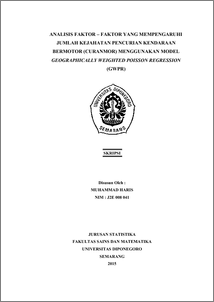# ANALISIS FAKTOR – FAKTOR YANG MEMPENGARUHI JUMLAH KEJAHATAN PENCURIAN KENDARAAN BERMOTOR (CURANMOR) MENGGUNAKAN MODEL GEOGRAPHICALLY WEIGHTED POISSON REGRESSION (GWPR)

HARIS, MUHAMMAD (2015) ANALISIS FAKTOR – FAKTOR YANG MEMPENGARUHI JUMLAH KEJAHATAN PENCURIAN KENDARAAN BERMOTOR (CURANMOR) MENGGUNAKAN MODEL GEOGRAPHICALLY WEIGHTED POISSON REGRESSION (GWPR). Undergraduate thesis, FSM Universitas Diponegoro.Preview
PDF
858Kb

## Abstract

Theft is an act taking someone else’s property, partially or entierely, with intention to have it illegally. Motor vehicle theft is one of the most highlighted crime type and disturbing the communities. Regression analysis is a statistical analysis for modeling the relationships between response variable and predictor variable. If the response variable follows a Poisson distribution or categorized as a count data, so the regression model used is Poisson regression. Geographically Weighted Regression (GWR) model is developed from global regression model where every parameter is counted for each observation location. Therefore, every observation location has different regression parameters. Geographically Weighted Poisson Regression (GWPR) is a local form of Poisson regression where data sampling location is prioritized. GWPR model is used for identifying the factors that influence the numbers of motor vehicles theft, either using a weighted gauss kernel function or bisquare kernel function. By using different weighting, the variables effecting the number of motor vehicle theft every Sub-District in the Semarang city is also different. Based on the value of Akaike Information Criterion (AIC) of Poisson regression and GWPR model, it is analyzed that GWPR model using a weighted fixed bisquare kernel function is the best model for analyzing the number of motor vehicles theft at every Sub-Districts in the Semarang city in 2012, because it has the smallest AIC value. This model has a precision of 88,81%. Key words : Motor Vehicle Theft, Geographically Weighted Poisson Regression, Kernel Gauss Function, Kernel Bisquare Function, Akaike Information Criterion

Item Type: Thesis (Undergraduate) H Social Sciences > HA Statistics Faculty of Science and Mathematics > Department of Statistics 47165 Mr Hasbi Yasin 22 Dec 2015 15:04 22 Dec 2015 15:04

Repository Staff Only: item control page